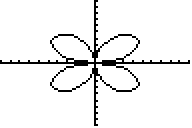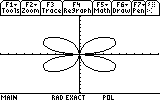# Knowledge Base

## Solution 12192: Graph of Certain Functions in Polar Mode Displays Negative Values on TI Graphing Calculators.

### In polar mode, why does the graph of certain functions display negative values?

When graphing certain functions in polar coordinates, can indeed be negative. For example, the graph of 6*sin(2q). represents the distance away from the origin (radius), and q represents the angle deviation from 0. If is negative, then the point is reflected over the origin. If q is positive, then the point rotates counter clockwise and if it's negative the point rotates clockwise. Each point has more than one way to describe it depending on which direction the rotation goes.

Most calculus books say that must be positive. The reason is that calculus deals with real-life type situations. In the real world, it doesn't make much sense to say that something has negative radius, so the negative radius is usually converted to a positive (which affects the q value). For example, the point (-(2), -p/4) is equal to ((2), 3p/4).

Notice that the difference between the two numbers is that
• The sign of r has changed from negative to positive.